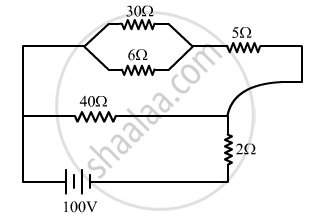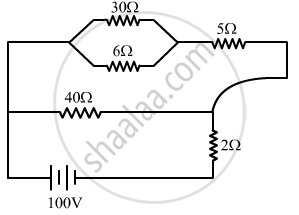Share

# A 100 V Battery is Connected to the Electric Network as Shown. If the Power Consumed in the 2 ω Resistor is 200 W, Determine the Power Dissipated in the 5 ω Resistor. - Physics

ConceptElectric Current

#### Question

A 100 V battery is connected to the electric network as shown. If the power consumed in the 2 Ω resistor is 200 W, determine the power dissipated in the 5 Ω resistor.#### Solution

Total current$I = \sqrt{\frac{P}{R}} = \sqrt{\frac{200}{2}} = 10A$

Equivalent circuit:Potential difference across AB = potential difference across CD

$\Rightarrow I_1 R_1 = I_2 R_2$

$\Rightarrow 10 I_1 - 40 I_2 = 0$

$\Rightarrow I_1 - 4 I_2 = 0 . . . (1)$

$\Rightarrow I_1 + I_2 = I$

$\Rightarrow I_1 + I_2 = 10 . . . (2)$

$\Rightarrow I_1 = 8A$

Power dissipated in the 5 Ω resistor = 5 $\times$  (current through 5 Ω resistor)2
∴ P = 320 W

Is there an error in this question or solution?

#### Video TutorialsVIEW ALL 

Solution A 100 V Battery is Connected to the Electric Network as Shown. If the Power Consumed in the 2 ω Resistor is 200 W, Determine the Power Dissipated in the 5 ω Resistor. Concept: Electric Current.
S